# Power Query – If Statements for Conditional Logic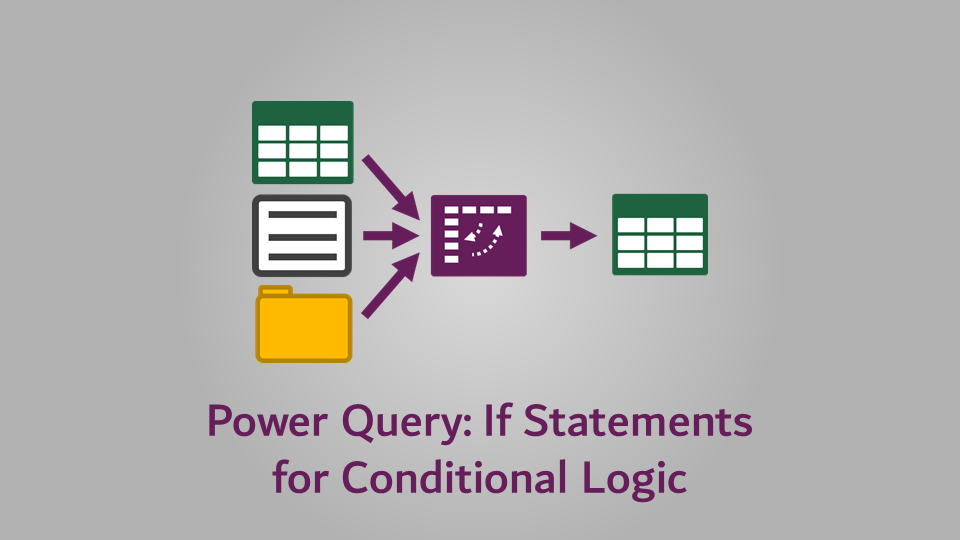In a previous post, we briefly looked at the if statement in Power Query, now we’re going to dig a bit deeper and understand how the Power Query if really works.

In Excel, IF is a core function, it’s one of the first ones we learn.  Last week, we looked at Functions in Power Query, but we didn’t cover a Power Query version of the IF function.  Surely there is an if function… right?  … well, kind of.

To use Power Query if logic, we need a programming based methodology, rather than tje function based methodology we find in Excel.  If you’ve ever programmed VBA or other languages, you will be familiar with this approach.  However, if you’re coming from a purely Excel world this may be new to you.

There are two options:

1. Using the conditional column feature for basic if logic
2. Writing M code for more advanced if logic.

We’ll cover both in this post.

I recommend you download the example file for this post.  Then you’ll be able to work along with examples and see the solution in action, plus the file will be useful for future reference.

The examples in this post use the file called Example 15 – If Function.xlsx

## The Example

The file contains just one Table.  Select any cell in that Table and click Data -> From Table/Range to load the data into Power Query.

In this example, we will be calculating the value assuming the following:

• Scenario 1: Sundays have an additional 10% premium (requires basic logic)
• Scenario 2: Sundays have an additional 10% premium, with two products (Tiger & Farmhouse Bloomer) also attracting a 5% discount on that day (requires advanced logic).

## Power Query If logic using a conditional column

### Scenario 1 – Sunday Premium

Select the Date column, then add a column containing the weekday by clicking Add Column -> Date -> Day -> Day Name.

Next, click Add Column -> Conditional ColumnEnter the following options on the Add Conditional Column dialog box:

If Day Name equals Sunday then 1.1 else 1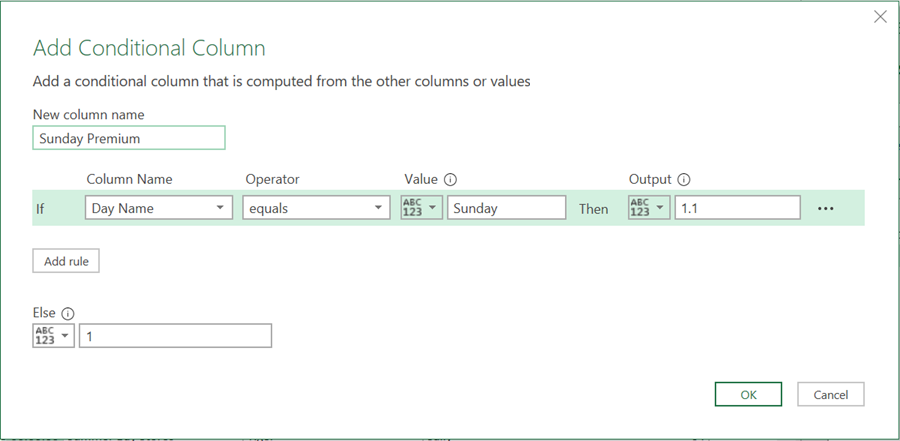Click OK to accept the formula.

In the scenario above, we have only used a few of the options available in the Conditional Column dialog box.

Other operators are available :

• Equals
• Does not equal
• Begins with
• Does not begin with
• Ends with
• Does not end with
• Contains
• Does not contain

In the Add Conditional Column dialog box, we can click the 123ABC button to insert column values, or parameters, instead of hardcoded values.

Also, we can create as many if statements as we want by clicking the Add rule button.  The if statements execute in turn, so where the first if statement is not triggered, it will move to the second, then the third, and so on.. However, if the first if statement is true, the remaining logic is skipped over.

Finally, let’s add a formula.  Click Add Column -> Custom Column to display the Custom Column dialog box.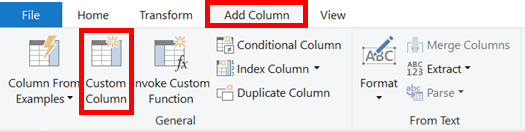Enter the following formula:

`=[Value] * [Sunday Premium]`

Call the formula Adjusted Value, then click OK.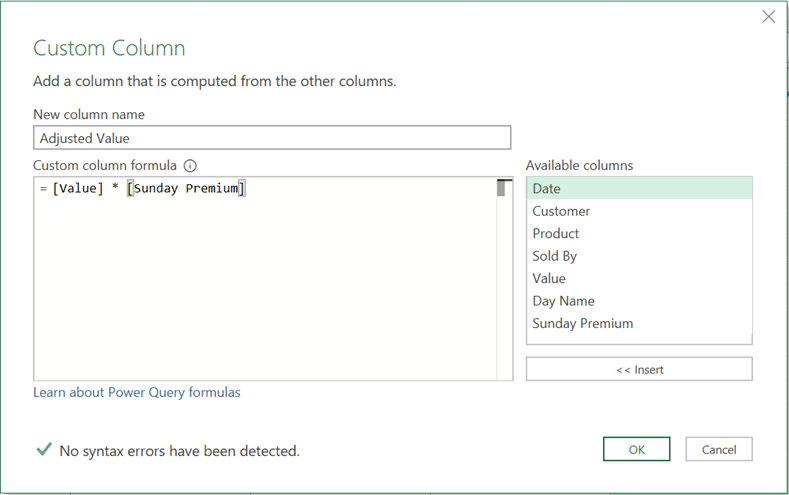Alternatively, for this last stage, you could have used a multiplication column Add Column -> Standard -> Multiply, but I prefer the Custom Column approach.

Hopefully you’ve seen that conditional logic is pretty easy… right.

### Scenario 2 – Sunday Premium & Product Discount

If you trying to use the Conditional Column with two or more conditions, things get tricky…very tricky.

I could go through a long list of transformations to illustrate how to achieve it with Scenario 2, but I’m not going to..  The Conditional Column feature is really for basic requirements; it doesn’t allow us to use AND or OR logic.

Instead, we are going to turn to option 2, which is writing the Power Query M code ourselves.  Don’t worry, I believe in you.  You can do this 🙂

## IF logic by writing the M code

Before launching into the examples, let’s take a few minutes to understand how the Power Query if works.

In an Excel world, the IF function would be written as:

`=IF( [thing] = "thing 2", [do this if true] , [do this if false] )`

So, if you are coming from an Excel world it might be easier to think of it like this:

• remove the opening and closing brackets
• change the first comma for then
• change the last comma for else
• change IF for if

This would create the following the M code

`= if [thing] = "thing 2" then [do this if true] else [do this if false]`

Once you’ve been through it a few times, you’ll get then hang of it.  It has the added advantage that it sounds like a standard English sentence, making it much easier to understand that the Excel equivalent.

To nest an if within another if we use the same if – then – else methodology, as shown below

```= if [thing to to test #1]  = "something else" then [do this if true]
else if [thing to to test #2]  = "something else" then [do this if true] else
[do this if false]```

There can be as many nested ifs as we want.  This works in a similar way to as adding new rules into a Conditional Column.

Using AND or OR logic is pretty simple too, we just use the words and or or in the code.  The code below demonstrates the use of and logic.

```= if [thing to to test #1]  = "something else"
and [thing to to test #2]  = "something else #2"
then [do this if true] else [do this if false]```

Where there is a mix of both and/or logic, brackets are required to ensure the logic evaluates in the correct order.

That’s covered the basics of if in Power Query, so it’s now time for the examples.

Start from scratch by loading the Table into Power Query again.  Then add the Week Day name column as we did in the example above.

### Scenario 1 – Sunday Premium

Add a Custom Column to the table by clicking Add Column  -> Custom ColumnUse the following formula:

`= if [Day Name] = "Sunday" then [Value] * 1.1 else [Value]`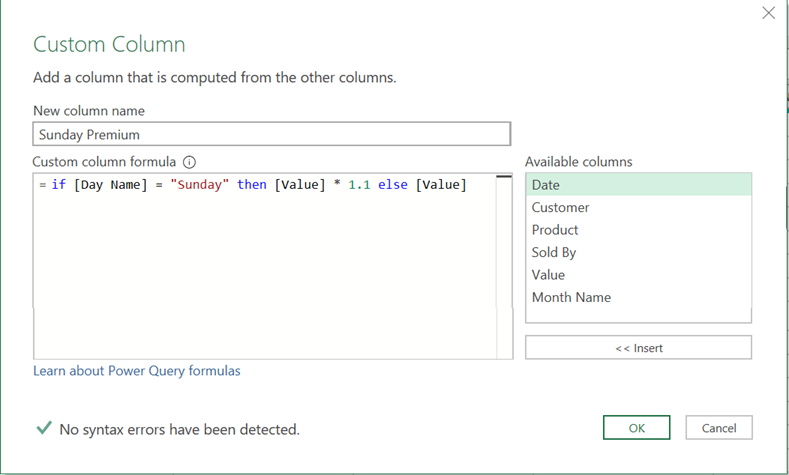Pay close attention to the words if, then and else, they are all lower case.  Power Query is case sensitive, so if we get this wrong, the formula will not work.

That’s it.  Click OK to add the formula.

### Scenario 2 – Sunday Premium & Product Discount

There are a few ways to write this formula.  Based on our data set, there are three possible results for this scenario:

• Sunday premium & product discount

Solution #1:

```= if [Day Name] = "Sunday" and ([Product] = "Tiger" or [Product] = "Farmhouse Bloomer") then
[Value] * 1.1 * 0.95
else if [Day Name] = "Sunday"
then [Value] * 1.1
else [Value]```We needed to include some brackets in here to ensure the formula executes in the correct order.  We want the or element to be calculated first, so we put this section in brackets.

Solution #2:

Here is another solution you could try.

```= if [Day Name] = "Sunday" then
if [Product] = "Tiger" or [Product] = "Farmhouse Bloomer" then [Value] * 1.1 * 0.95
else [Value] * 1.1
else [Value]```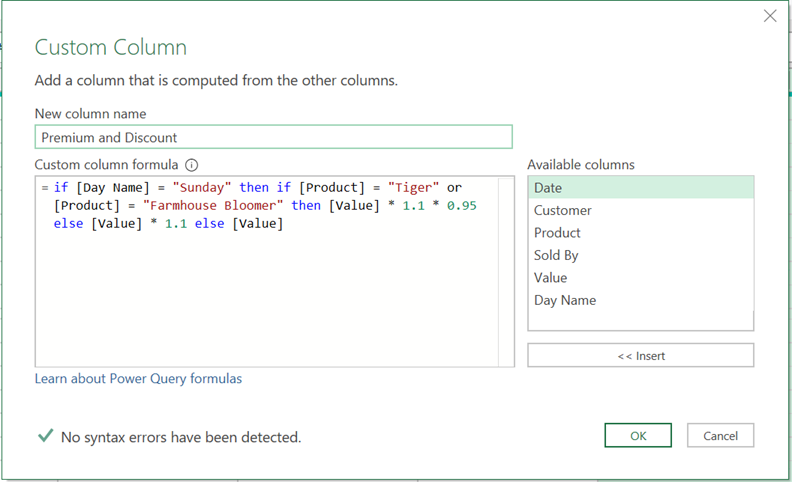## Conclusion

Conditional logic using an if statement in Power Query is different to Excel.  However, it’s not difficult to understand, so all it needs is practice.Get our FREE VBA eBook of the 30 most useful Excel VBA macros.
Automate Excel so that you can save time and stop doing the jobs a trained monkey could do.

Email Address * First Name *

Don’t forget:

I’m guessing the examples in this post didn’t exactly meet your situation.  We all use Excel differently, so it’s impossible to write a post that will meet everybody’s needs.  By taking the time to understand the techniques and principles in this post (and elsewhere on this site) you should be able to adapt it to your needs.

But, if you’re still struggling you should:

1. Read other blogs, or watch YouTube videos on the same topic.  You will benefit much more by discovering your own solutions.
2. Ask the ‘Excel Ninja’ in your office.  It’s amazing what things other people know.
3. Ask a question in a forum like Mr Excel, or the Microsoft Answers Community.  Remember, the people on these forums are generally giving their time for free.  So take care to craft your question, make sure it’s clear and concise.  List all the things you’ve tried, and provide screenshots, code segments and example workbooks.
4. Use Excel Rescue, who are my consultancy partner.   They help by providing solutions to smaller Excel problems.

What next?
Don’t go yet, there is plenty more to learn on Excel Off The Grid.  Check out the latest posts:

## 15 thoughts on “Power Query – If Statements for Conditional Logic”

1.Isaac says:

Hello. I have a question. I would like to erase a column if it is empty. Is there a way to construct an instruction like the following in a table step?:

=If then #previous_table else Table.RemoveColumn(#”previous_table”, “column_to_check”)

where the code in is the code I would like to see how to build. Thank you.

•Excel Off The Grid says:

There are a couple of options for working in the Advanced Editor. I’m sure there are more, but these are the ones I’ve just thought of.

#”Jump to another step” = if Table.RowCount(Table.SelectRows(#”PreviousStep”, each [RemoveThisCol] = null)) = 0 then #”PreviousStep” else #”PreviousPreviousStep”

Remove a column of the condition is true/false:
#”Remove Col” = if Table.RowCount(Table.SelectRows(#”PreviousStep”, each [Headcount] null)) = 0 then #”PreviousStep” else Table.RemoveColumns(#”PreviousStep”,{“Headcount”})

2.Mark says:

Hi,
I have just started to use power query and find it really useful but as I am still learning I’m not sure if I can achieve the same results that I can with an excel formula.

I need to find numbers with in long blocks of text descriptions.
In excel I currently use a formula that returns a simple yes or no if a cell contains any number in the text description column. =IF(COUNT(FIND({0,1,2,3,4,5,6,7,8,9},A1))>0, “Yes”, “No”)

Is there a way to do the same using IF logic in power query?

•Excel Off The Grid says:

Yes, you can, try this when using the Add Column feature. Replace “myColumnName” with the name of your column.

=if Text.Select([myColumnName], {“0”..”9″}) <> “” then “Yes” else “No”

•Mark says:

Thank you so much, I’d have never figured that out.

3.Huibert says:

Hello,

How can I apply bigger than but smaller than formules in a power query?

•Excel Off The Grid says:

It’s as simple as using the word and between the conditions:

`if [TestField] > 2 and [TestField] < 7 then "3 to 6" else "other values"`
4.Barry Mahbouby says:

I am only just learning to use Excel Power Query 2016 and I need to filter a column of dates to be equal to today or less than today but cannot see any option to doing this.

5.Indra Maulana says:

Hi,
Is it possible to get the conditional logic using if statement from parameter that resides in a csv/text/excel files? The criteria / multiple criteria will be read by power query based what listed in it. Why do I need to do this because the criteria that need to be evaluate is more than 100 and can dinamically change over time.
Is there a way to achieve that in excel power query? Thank you

6.Pablo says:

Hi,
how did you get the different color fonts on the query editor (blue for conditionals, red for values, green for numbers)? Mine’s all plain black.
Thank you

•Excel Off The Grid says:

This was rolled out by Microsoft as an update within the Office 365 subscription.

7.